# Ensemble Test with Gaussian using RooFit

I would like to perform a sort of ensemble test by fitting a gaussian PDF to gaussian generated data. I have coded up something but for some reason my pull distributions are all off. The following with code is what I have done.

1: Generate a gaussian distribution with a mean of 100 and a width of 20 (I should specifiy that nentries = 1000 here)

``````TH1* genMC(TRandom3 *rng, TH1 *input) {
if(input == 0x0) return 0x0;

// Clone the histogram
TH1 *output = (TH1*) input->Clone();

long nentries = output->GetEntries();

// Empty it out
output->Reset();

for(long i = 0; i < nentries; i++) {
output->Fill(rng->Gaus(100, 20));
}

return(output);
}``````

2: I then pass this into a function that does the fitting using RooFit

``````Result doFit(TH1 *input_sideband, TH1 *input_signal, bool saveme) {
Result res;

double signal_region_count = input_signal->Integral(input_signal->FindBin(0), input_signal->FindBin(300));
double sideband_region_count = input_sideband->Integral(input_sideband->FindBin(0), input_sideband->FindBin(300));

RooRealVar gauss_mean("mean", "mean", 70, 50, 150);
RooRealVar gauss_width("width", "width", 15, 0, 30);
RooGaussian thresh("gauss", "Signal PDF", x, gauss_mean, gauss_width);

RooDataHist data_sideband("data", "Yhea", x, input_sideband);
x.setRange("fit", 0, 300);

RooPlot *frame = 0x0;

frame = x.frame();
data_sideband.plotOn(frame, MarkerColor(1), LineColor(1));
data_sideband.statOn(frame);
// Generate the extended likelyhood stuff
RooRealVar nbkg("nbkg", "Number of Background Events", 100, 0, 10000000);
RooAddPdf bkgModel("BkgModel", "Background Model", RooArgList(thresh), RooArgList(nbkg));

// Do the fit to the sidebands
RooFitResult *fitResult_Sideband = bkgModel.fitTo(data_sideband, Extended(kTRUE), Range("fit"), Verbose(kTRUE), Save(kTRUE), Minos(kFALSE), PrintLevel(3\$

bkgModel.plotOn(frame, LineColor(kRed), LineStyle(2), Normalization(nbkg.getVal(), RooAbsReal::NumEvent));
bkgModel.paramOn(frame, ShowConstants(kTRUE), Label("Fit Parameters"), Format("NEU", AutoPrecision(1)));

int status = fitResult_Sideband->status();
int covQual = fitResult_Sideband->covQual();
cout << "The sideband fit status was: " << status << " with a covariance quality code of: " << covQual << endl;

res.obs               = sideband_region_count;
res.obs_err           = sqrt(res.obs);
res.exp               = nbkg.getVal();
res.exp_err           = nbkg.getError();
res.delta             = res.obs - res.exp;
res.pull              = res.delta / res.exp_err;
res.side_fit_status   = fitResult_Sideband->status();
res.side_cov_status   = fitResult_Sideband->covQual();
res.mean              = gauss_mean.getVal();
res.mean_err          = gauss_mean.getError();
res.width             = gauss_width.getVal();
res.width_err         = gauss_width.getError();

return(res);
}``````

I do the above 1000 times and the Results are then saved into a root file for viewing. Now, I would expect to get a nice pull distribution centered at 0 with a width of 1. I do not. I have attached the pull distribution to this post so that you can see it. I have also included as an attachment the full code with all of its spaghetti glory. Is this a by product of me using the extended likelihood technique? Thanks for any help on this.

Justace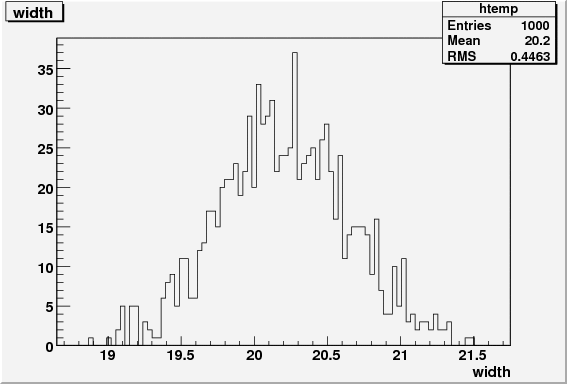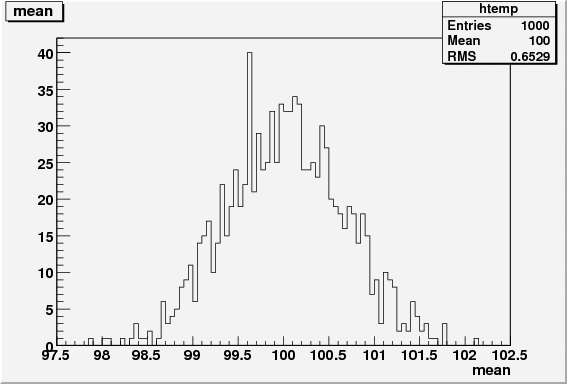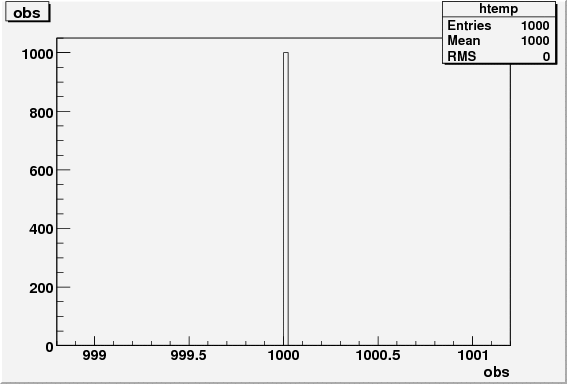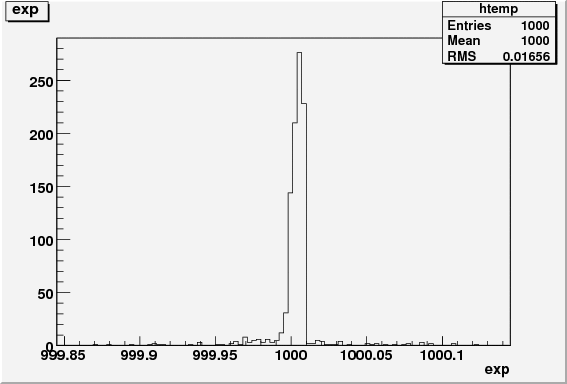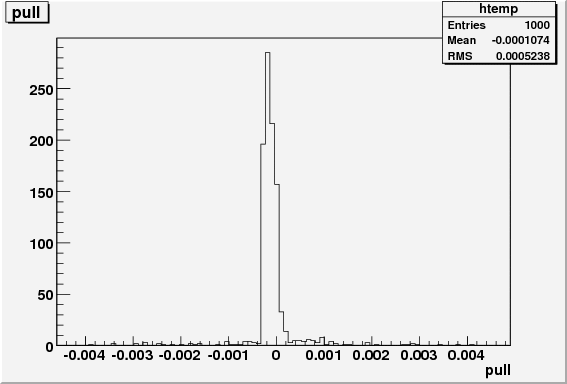code.tar.gz (4.95 KB)

Hi Justace,

If the problem is in the pull distribution of your nsig, this is indeed related to extended likelihoods. If you want to generate correct pull distribution for the event count you need to generate your input samples with a Poisson fluctuation, i.e. instead of generation exactly 1000 events for each experiment you should generation gRandom->Poisson(1000)
events,

Wouter

PS: RooFit has a built-in tool to manage such toy studies RooMCStudy, see
\$ROOTSYS/tutorials/roofit/rf801_mcstudy.C (ROOT>=5.21-02)
root.cern.ch/viewvc/trunk/tutori … iew=markup